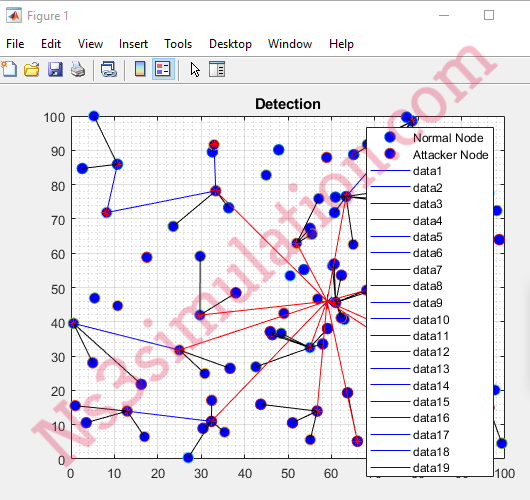Ns3 Projects for B.E/B.Tech M.E/M.Tech PhD Scholars.  Phone-Number:9790238391   E-mail: ns3simulation@gmail.com

# How Do I Calculate Energy Consumed by the Sensor or Nodes in Matlab for Wireless Sensor Network

Initially, we have to create the process file along with the file extension .m and writing the Matlab source code to call the sensor to calculate the energy.

``` for i=1:1:n if ( S(i).type=='N' && S(i).E>0 ) % min_dis = sqrt( (S(i).xd-S(n+1).xd)^2 + (S(i).yd-S(n+1).yd)^2 ); min_dis = INFINITY; if(cluster -1 >= 1) min_dis_cluster = 1; for c = 1:1:cluster - 1 temp = sqrt( (S(i).xd - C(c).xd)^2 + (S(i).yd - C(c).yd)^2 ); if ( temp < min_dis ) min_dis = temp; min_dis_cluster = c; end S(i).E = S(i).E - ETX * ctrPacketLength; end min_dis; if (min_dis > do) S(i).E = S(i).E - ( ETX*(ctrPacketLength) + Emp * ctrPacketLength*( min_dis * min_dis * min_dis * min_dis)); S(i).E = S(i).E - ( ETX*(packetLength) + Emp*packetLength*( min_dis * min_dis * min_dis * min_dis)); else S(i).E = S(i).E - ( ETX*(ctrPacketLength) + Efs*ctrPacketLength*( min_dis * min_dis)); S(i).E = S(i).E - ( ETX*(packetLength) + Efs*packetLength*( min_dis * min_dis)); end S(i).E = S(i).E - ETX*(ctrPacketLength); if(min_dis > 0) S(C(min_dis_cluster).id).E = S(C(min_dis_cluster).id).E - ( (ERX + EDA)*packetLength ); S(C(min_dis_cluster).id).E = S(C(min_dis_cluster).id).E - ERX *ctrPacketLength ; if (min_dis > do) S(C(min_dis_cluster).id).E = S(C(min_dis_cluster).id).E - ( ETX*(ctrPacketLength) + Emp * ctrPacketLength*( min_dis * min_dis * min_dis * min_dis)); else S(C(min_dis_cluster).id).E = S(C(min_dis_cluster).id).E - ( ETX*(ctrPacketLength) + Efs * ctrPacketLength*( min_dis * min_dis)); end PACKETS_TO_CH(r+1) = n - dead - cluster + 1; end S(i).min_dis = min_dis; S(i).min_dis_cluster = min_dis_cluster; end end end ```

Then, we have to run the sensor network projects in Matlab through right clicking on the main file and selecting the Run option as mentioned in the following.Following that, we have highlighted the result of running main file in the sensor network through the utilization of Matlab.Additionally, we have highlighted the result about the energy consumption calculation in the graphical representation.Reach us to aid more!!!!